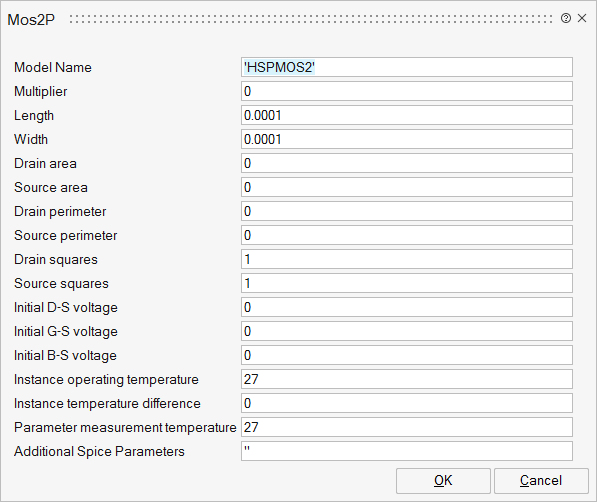# Mos2P

Level 2 Grove-Frohman Mosfet Model (NMOS)Analog/Basic

## Description

This is a MOS Level 2 (Grove-Frohman Mosfet Model)

The equations of the MOS level 2 give the evaluation of the current IDS between the drain and the source as a function of VD, VG and VS.

The device operation is divided into three regions:

- cut-off (Vgs <= Vth)

- linear (0 <= Vds <= Vgs-Vth)

- saturated (0 <= Vgs-Vth <= Vds)

The second order effect is taken into account (channel length modulation)

Equations for the MOS2N device

- when Vgs <= Vth then Ids=0

- when 0 <= Vds <= Vgs-Vth then the equations below apply:## ParametersNameLabelDescriptionData TypeValid Values

sp_mname

Model Name

Name of the Mos model

String

sp_m

Multiplier

Multiplier for setting m mosfets in parallel

Scalar

sp_l

Length

Channel length (meter)

Scalar

sp_w

Width

Channel width (meter)

Scalar

Drain area

Drain area (square meter)

Scalar

sp_as

Source area

Source area (square meter)

Scalar

sp_pd

Drain perimeter

Drain perimeter (square meter)

Scalar

sp_ps

Source perimeter

Source perimeter (square meter)

Scalar

sp_nrd

Drain squares

Equivalent number of squares of the drain diffusion

Scalar

sp_nrs

Source squares

Equivalent number of squares of the source diffusion

Scalar

sp_icvds

Initial D-S voltage

Initial drain source voltage (volts)

Scalar

sp_icvgs

Initial G-S voltage

Initial gate source voltage (volts)

Scalar

sp_icvbs

Initial B-S voltage

Initial bulk source voltage (volts)

Scalar

sp_temp

Instance operating temperature

The temperature at which the mosfet is operating (degrees Celsius)

Scalar

sp_dtemp

Instance temperature difference

Temperature variation relative to the simulation temperature (degrees Celsius)

Scalar

sp_tnom

Parameter measurement temperature

Scalar

sp_Spice

For more details, see Additional Spice Parameters.

String

## Ports

NameTypeDescriptionIO TypeNumber

Drain

implicit

1

Gate

implicit

2

Source

implicit

3

Bulk

implicit

4